# 一类对称碰撞系统的间歇混沌控制方法 ∗

(湖南大学 机械与运载工程学院， 长沙 410082)

## 1 原系统的动力学分析

### 1 . 1 分段运动微分方程以及所对应的通解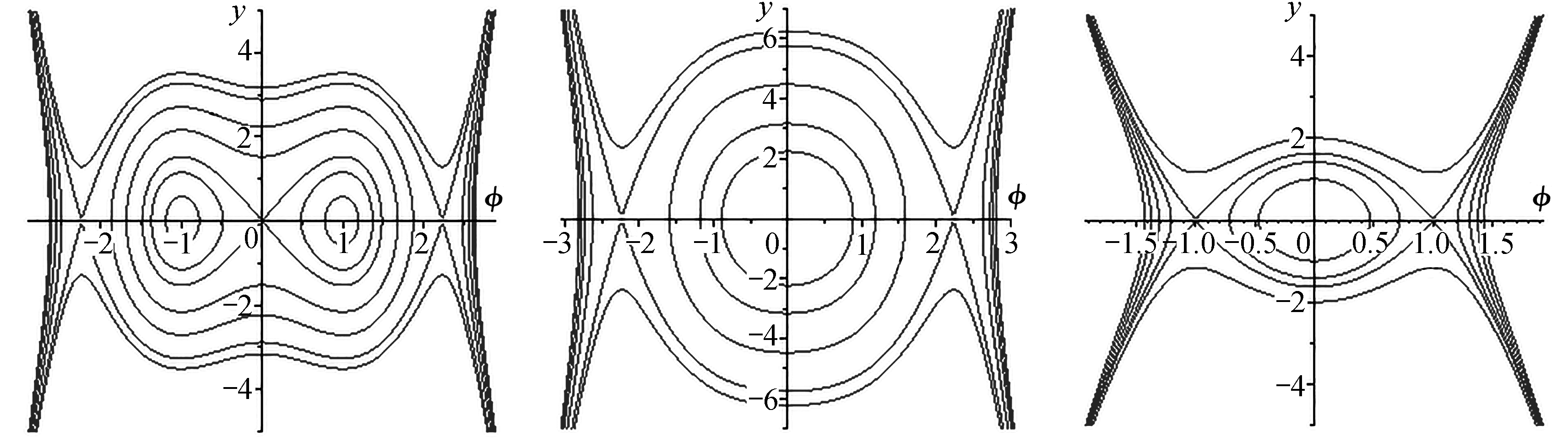Fig. 1 The dynamic model for a 2-DOF system under elastic symmetric impact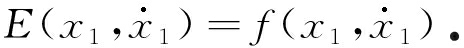t 0 t t 1 t 2 t t 3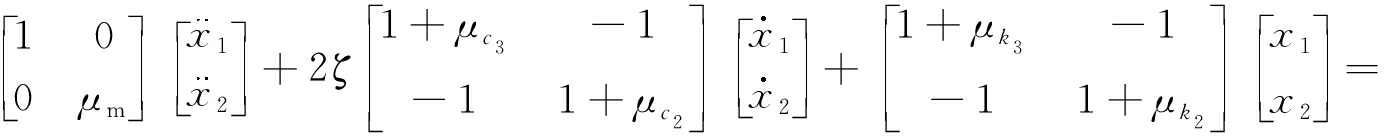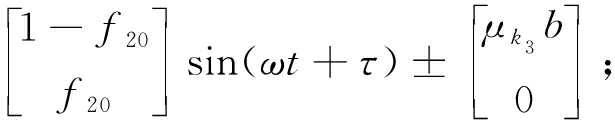(1)

t 1 < t t 2 t 3 < t t 4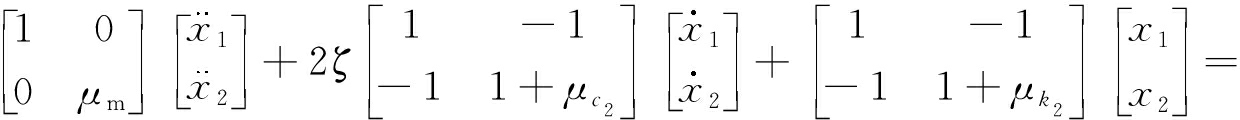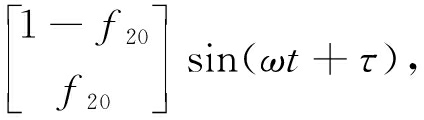(2)

μ m = M 2 / M 1 , μ c 2 = C 2 / C 1 , μ c 3 = C 3 / C 1 ,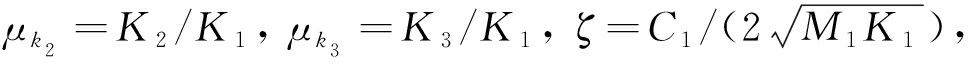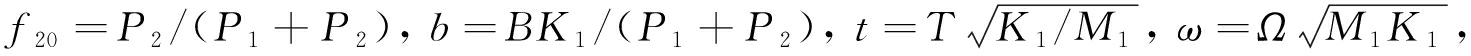x i = X i K 1 /( P 1 + P 2 ), i =1,2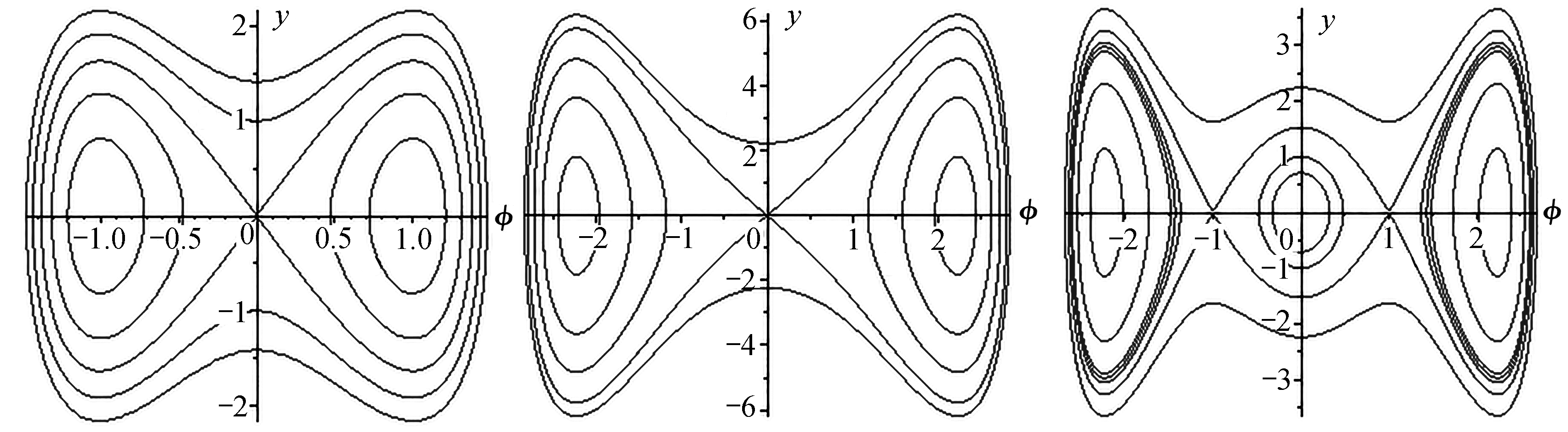Fig. 2 Boundary conditions

1) 通过模态叠加法可以求得以上各阶段方程所对应的通解,当 t 0 t t 1 t 2 t t 3 时，有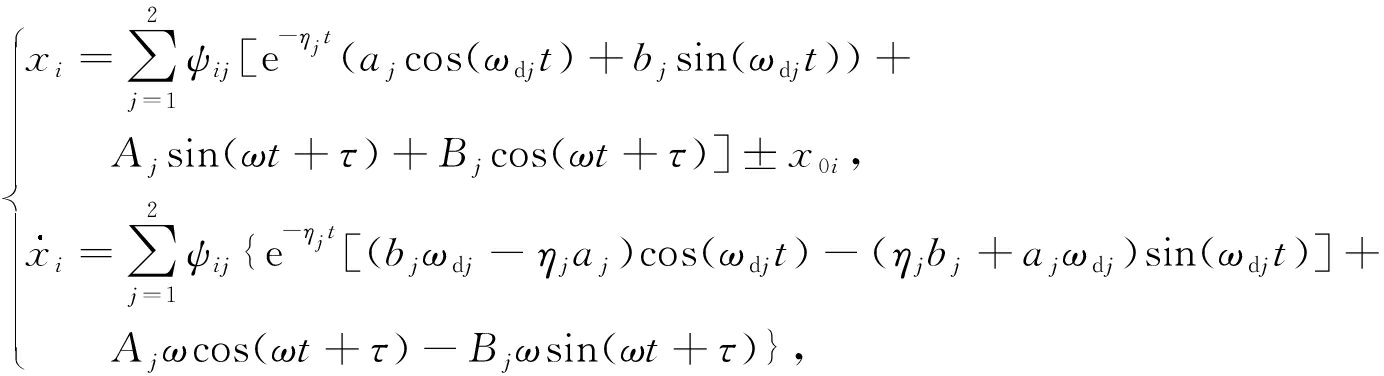(3)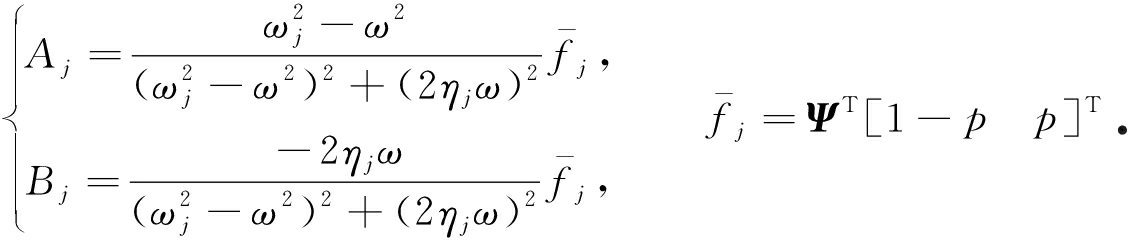2) 当 t 1 < t t 2 t 3 < t t 4 时，有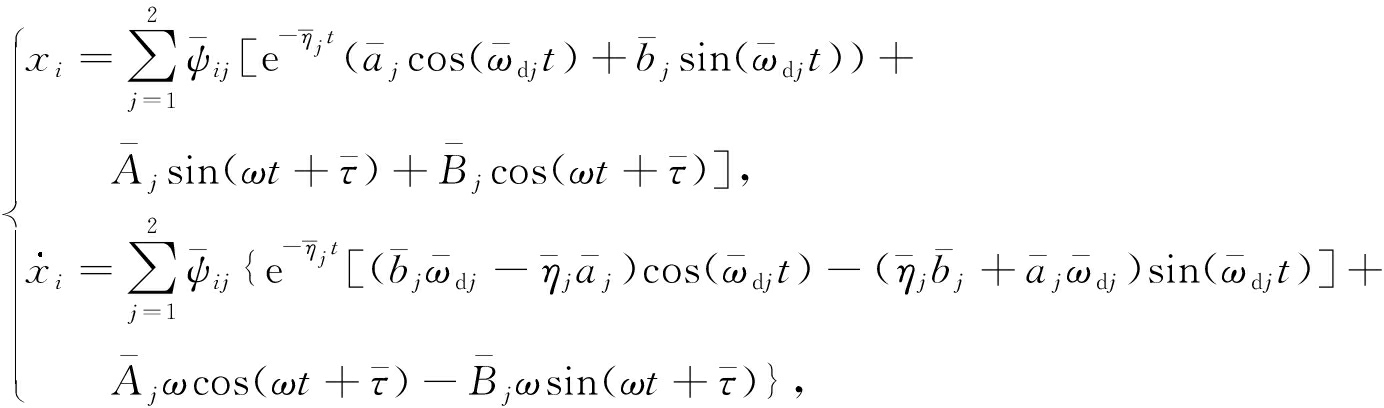(4)

### 1 . 2 Poincar é 映射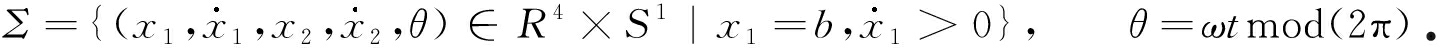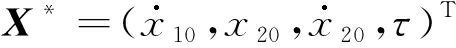表示系统在Poincaré截面上的周期 n -1不动点,将系统运动过程分为4个阶段，可分别建立相应的Poincaré映射：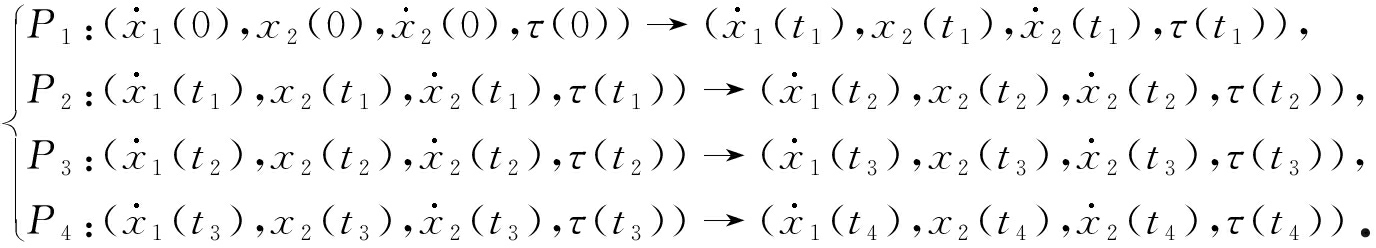(5)

P 1 , P 2 , P 3 , P 4 分别代表 P 1 , P 2 , P 3 , P 4 映射所对应的矩阵，于是系统的Poincaré映射 P 可以表示为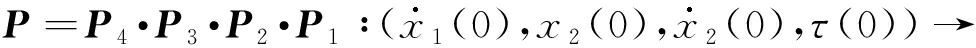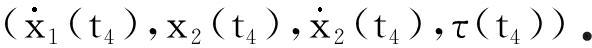(6)

## 2 控制系统的运动分析

### 2 . 1 控制系统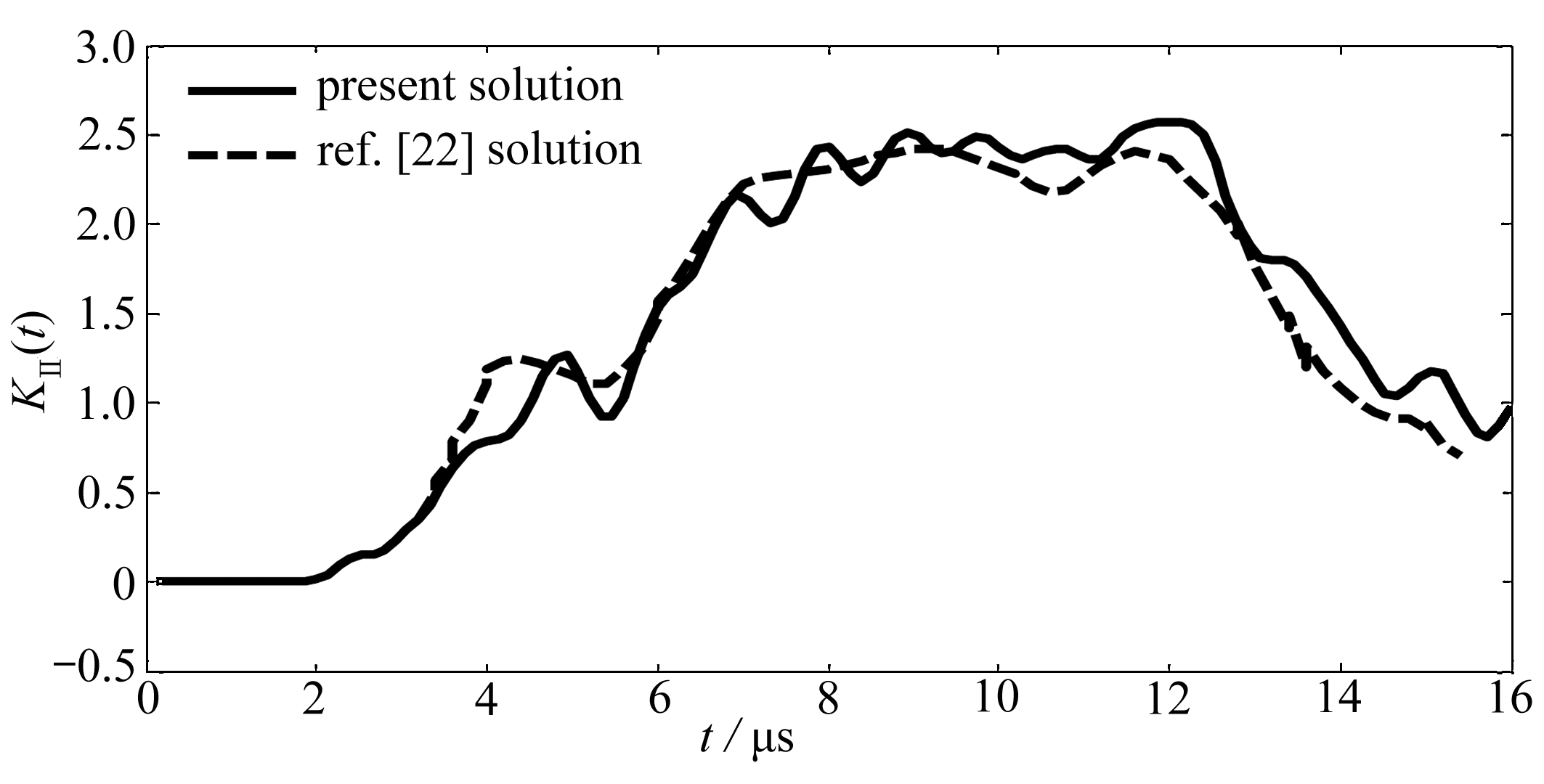Fig. 3 The location diagram with the control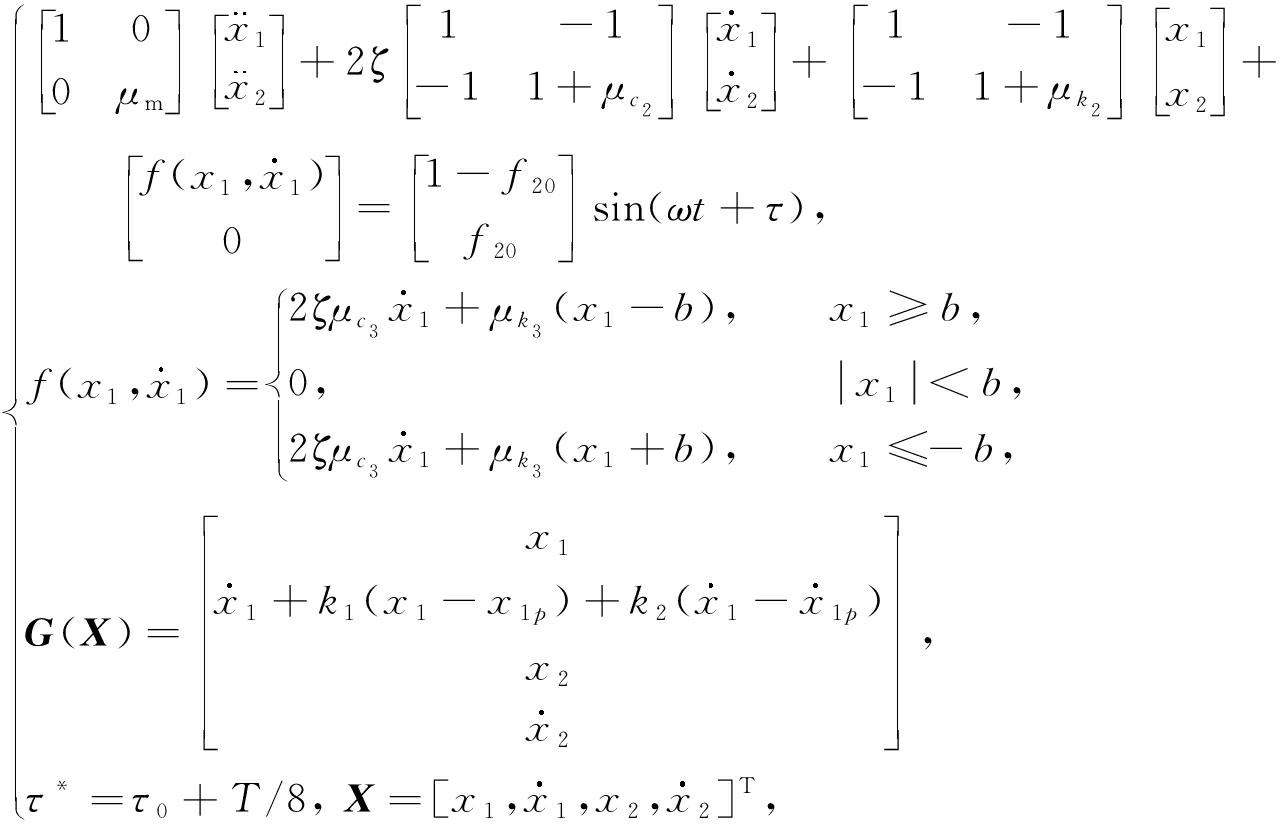(7)

P α = P 5 · P 4 · P 3 · G · P 2 · P 1

### 2 . 2 混沌控制的显式准则

P ( k 1 , k 2 ) ( λ )= m 0 ( k 1 , k 2 ) λ 4 + m 1 ( k 1 , k 2 ) λ 3 + m 2 ( k 1 , k 2 ) λ 2 + m 3 ( k 1 , k 2 ) λ +

m 4 ( k 1 , k 2 ), m n ( k 1 , k 2 )>0, m 0 ( k 1 , k 2 )=1, n =1,2,3,4

(8)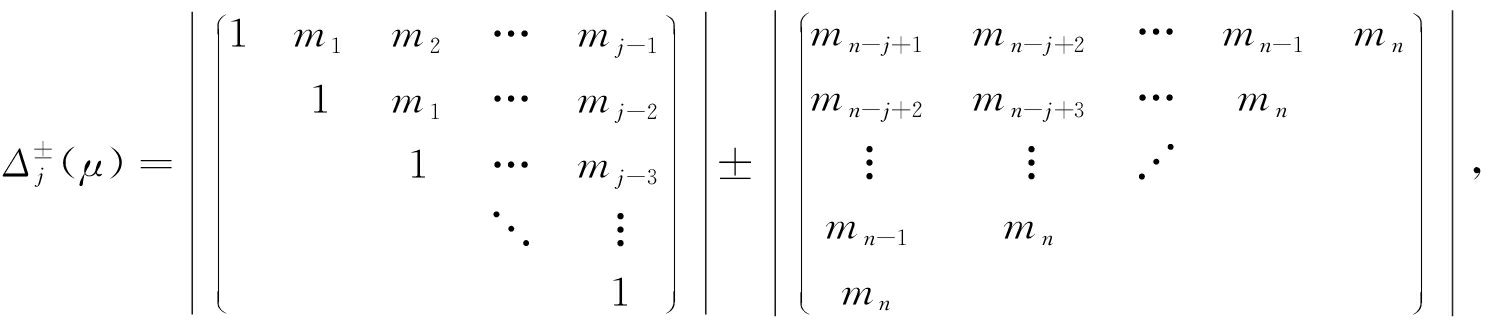j =1,2,…, n

(9)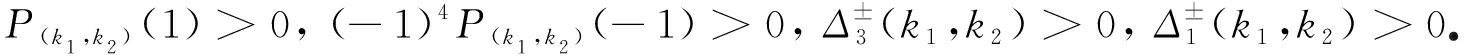(10)

1+ m 1 + m 2 + m 3 + m 4 >0,

(11a)

1- m 1 + m 2 - m 3 + m 4 >0,

(11b)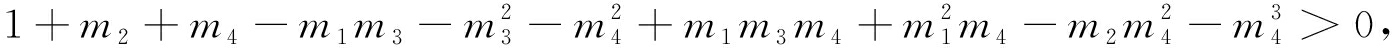(11c)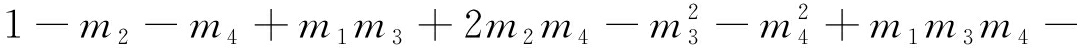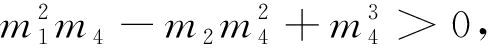(11d)

1+ m 4 >0,

(11e)

1- m 4 >0

(11f)

## 3 数 值 模 拟

### 3 . 1 原系统的数值模拟

μ m =2, μ k 2 =4, μ k 3 =6, μ c 2 =4, μ c 3 =6, p =0.01, ζ =0.01, b =0.1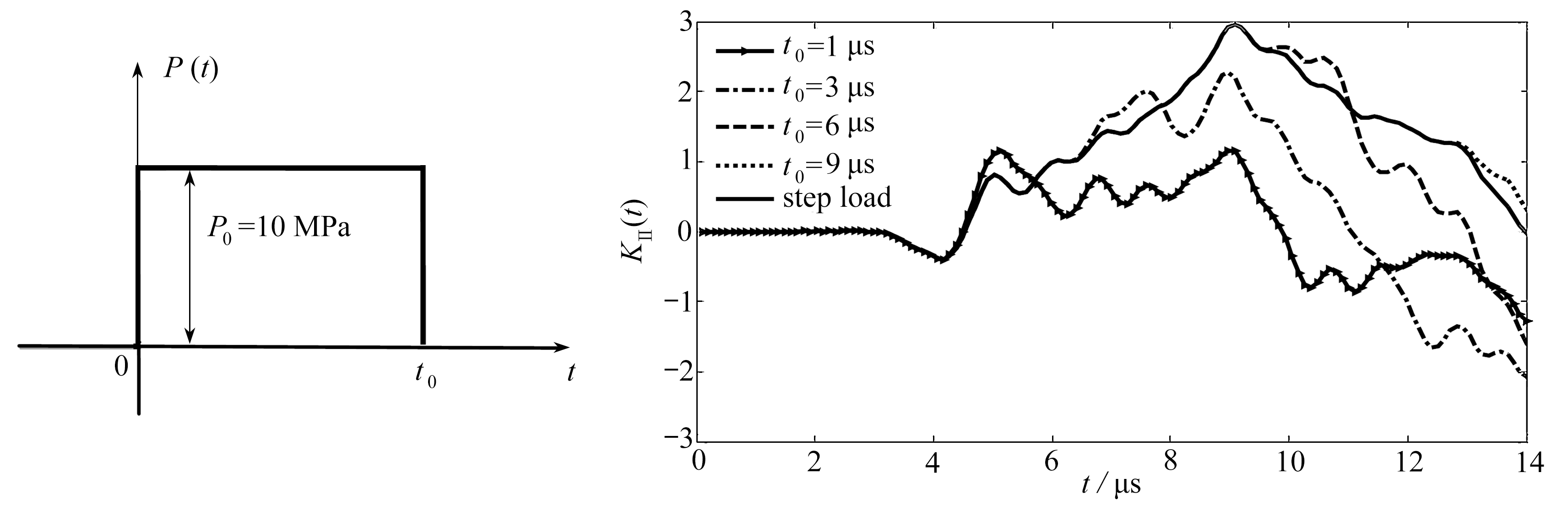Fig. 4 Global bifurcations of the original system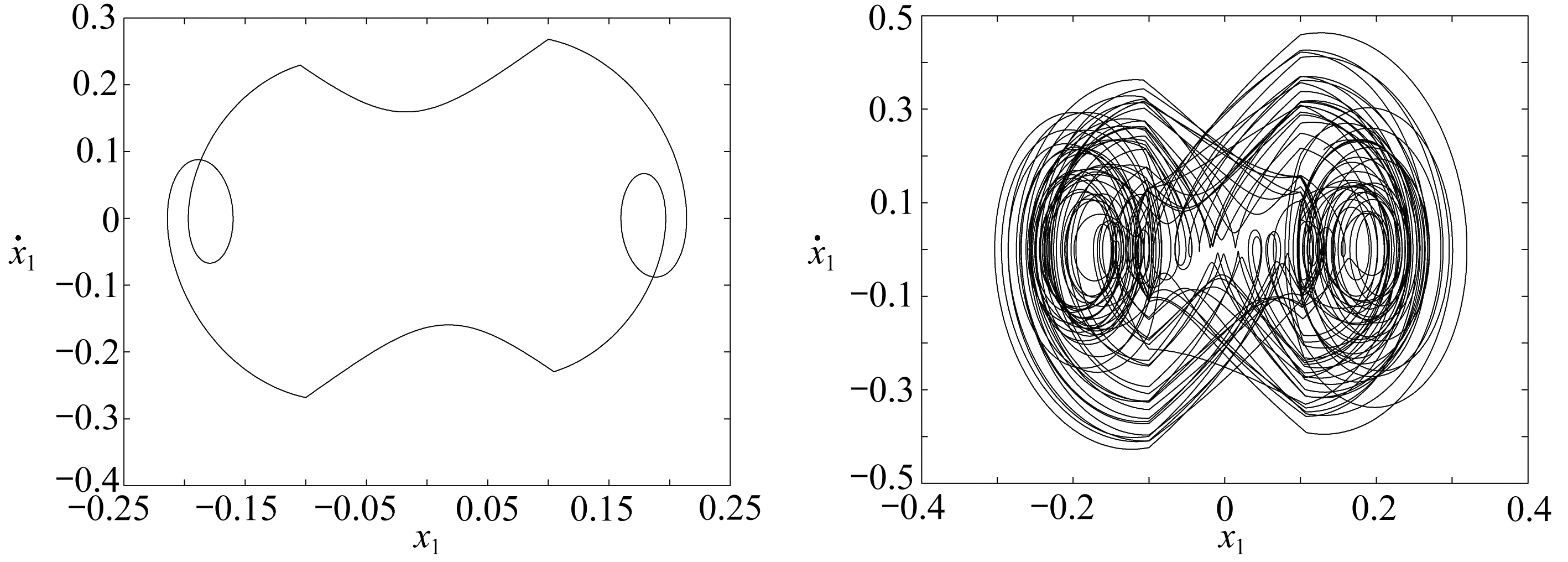(a) 对称双碰周期二(ω=0.72)(b) 混沌(ω=0.57)
(a) Symmetric impact with 2 cycles(ω=0.72)(b) Chaos (ω=0.57)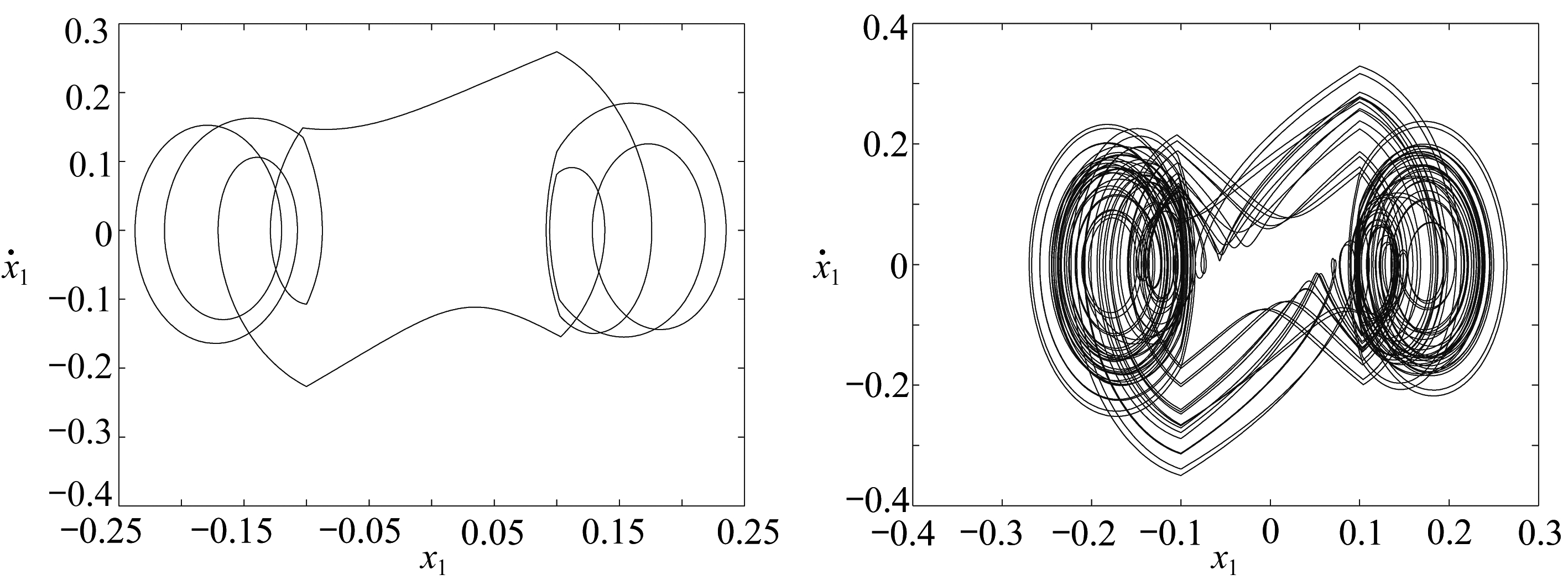(c) 稳定的多周期(ω=0.37)(d) 混沌(ω=0.32)
(c) Stable multiple cycles (ω=0.37)(d) Chaos (ω=0.32)

Fig. 5 The phase diagrams corresponding to the differentωvalues

λ 1 =8.738 2, λ 2 =0.040 1,

λ 3,4 =-0.213 7±0.717 3i,

λ 1 =8.738 2, λ 2 =0.040 1,

λ 3,4 =0.748 5

### 3 . 2 受控系统的数值模拟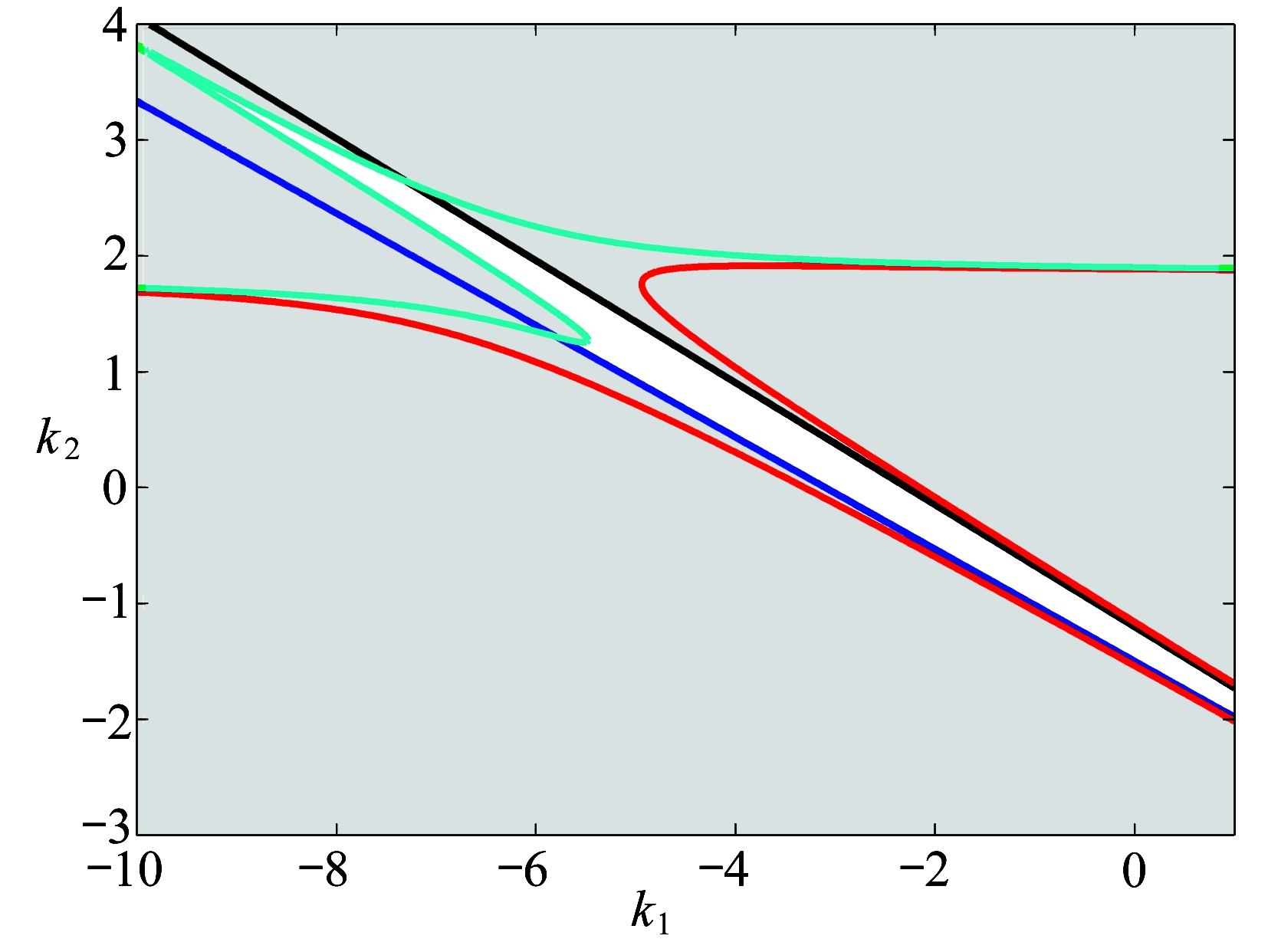Fig. 6 The bifurcation diagram of control parameters

λ 1,2 =-0.031 75±0.483 69i, λ 3,4 =-0.205 69±0.735 88i,

λ 1,2 =0.484 74, λ 3,4 =0.764 08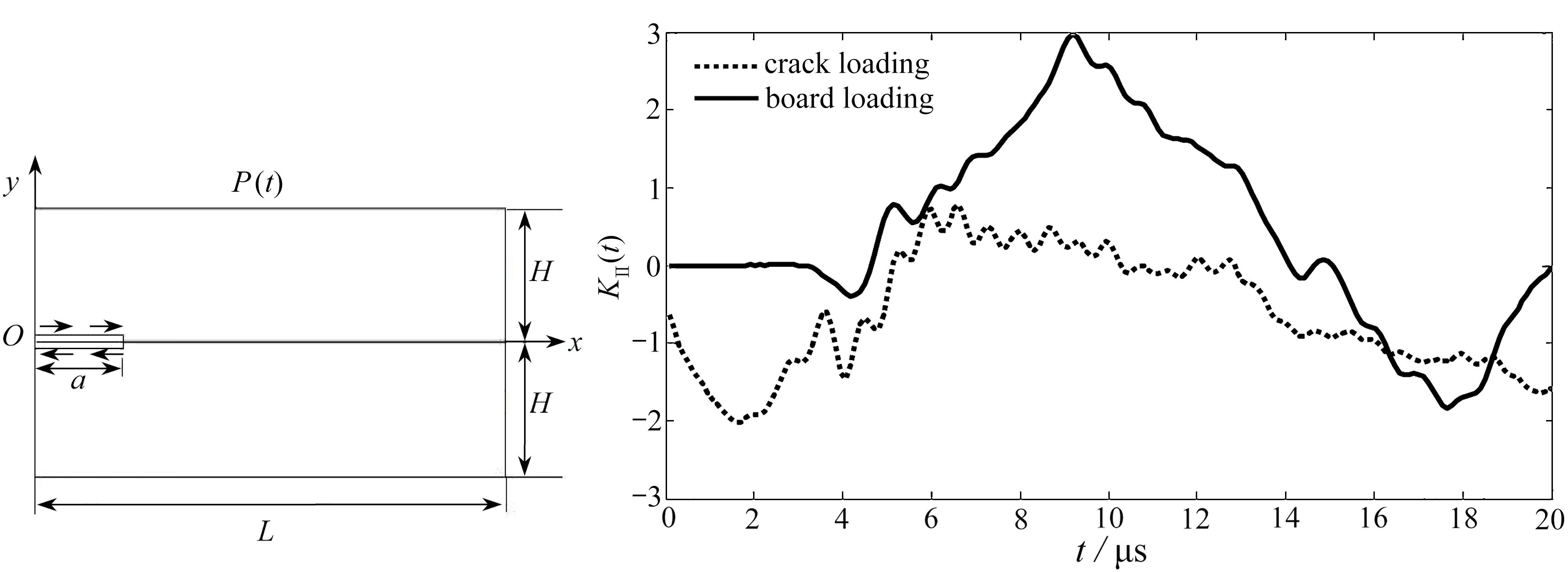(a) 控制前的混沌运动(b) 控制后的周期运动
(a) Chaotic motion without control (b) Controlled periodic motion

Fig. 7 The phase diagrams without and with control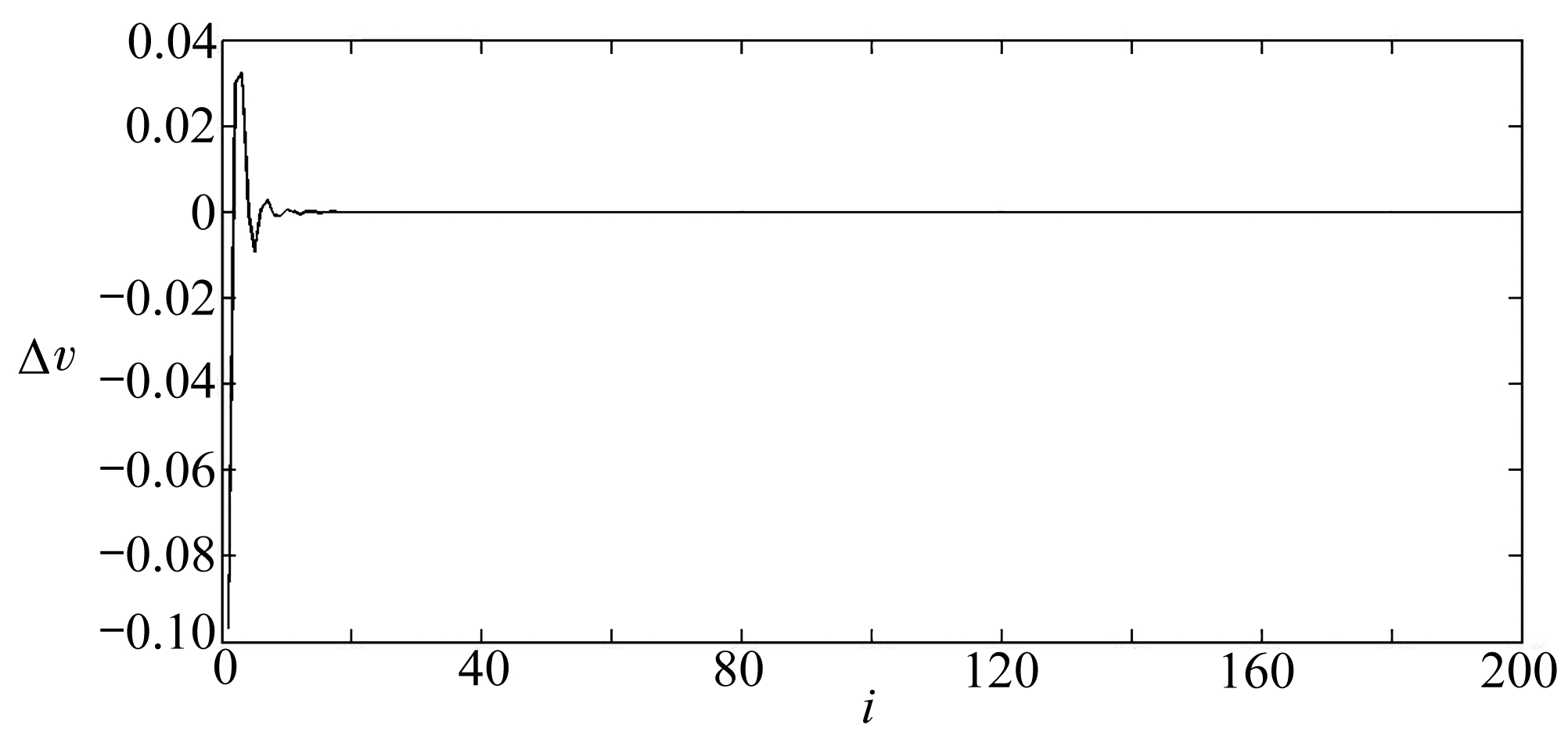Fig. 8 The control input curve with time

## 4 结 论

 OTT E, GREBOGI C, YORKE J. Controlling chaos[J]. Physical Review Letters , 1990, 64 (11): 1196-1199.

 杨凌, 刘曾荣. OGY方法的改进及证明[J]. 应用数学和力学, 1998, 19 (1): 1-7.(YANG Ling, LIU Zengrong. An improvement and proof of OGY method[J]. Applied Mathematics and Mechanics , 1998, 19 (1): 1-7.(in Chinese))

 张晓明, 彭建华, 张入元. 利用线性可逆变换增强延迟反馈方法控制混沌的有效性[J]. 物理学报, 2005, 54 (7): 3019-3026.(ZHANG Xiaoming, PENG Jianhua, ZHANG Ruyuan. Improving the efficiency of time-delayed feedback control of chaos through linear invertible transform[J]. Acta Physica Sinica , 2005, 54 (7): 3019-3026.(in Chinese))

 马莉. 一类双自由度含间隙振动系统的混沌碰撞运动及控制[J]. 兰州交通大学学报(自然科学版), 2007, 26 (6): 136-139.(MA Li. Controlling chaotic motions for a two-degree-of-freedom vibratory system with a clearance[J]. Journal of Lanzhou Jiaotong University ( Natural Sciences ), 2007, 26 (6):136-139.(in Chinese))

 李彬, 郝鹏, 孟增, 等. 基于改进自适应混沌控制的逆可靠度分析方法[J]. 应用数学和力学, 2017, 38 (9): 979-987.(LI Bin, HAO Peng, MENG Zeng, et al. An improved adaptive chaos control method for inverse reliability analysis[J]. Applied Mathematics and Mechanics , 2017, 38 (9):979-987.(in Chinese))

 吕小红. 外加正弦力抑制小型打桩机的分岔与混沌[J]. 兰州交通大学学报, 2015, 34 (4): 146-150.(LÜ Xiaohong. Bifurcations and chaos of small pile driver controlled by external sine force[J]. Journal of Lanzhou Jiaotong University , 2015, 34 (4): 146-150.(in Chinese))

 吕小红, 朱喜锋, 罗冠炜. 含双侧约束碰撞振动系统的OGY混沌控制[J]. 机械科学与技术, 2016, 35 (4):531-534.(LÜ Xiaohong, ZHU Xifeng, LUO Guanwei. Chaos control of a vibro-impact system with two-sided constraints based on OGY method[J]. Mechanical Science and Technology for Aerospace Engineering , 2016, 35 (4): 531-534.(in Chinese))

 朱喜锋, 罗冠炜. 两自由度含间隙弹性碰撞系统的颤碰运动分析[J]. 振动与冲击, 2015, 34 (15): 195-200.(ZHU Xifeng, LUO Guanwei. Chattering-impact motion of a 2-DOF system with clearance and soft impacts[J]. Journal of Vibration and Shock , 2015, 34 (15): 195-200.(in Chinese))

 张惠, 丁旺才, 李飞. 两自由度含间隙和预紧弹簧碰撞振动系统动力学分析[J]. 工程力学, 2011, 28 (3): 209-217.(ZHANG Hui, DING Wangcai, LI Fei. Dynamics of a two-degree-of-freedom impact system with clearance and pre-compressed spring[J]. Engineering Mechanics , 2011, 28 (3): 209-217.(in Chinese))

 LIU Yang, CHAVEZ J P. Controlling coexisting attractors of an impacting system via linear augmentation[J]. Physica D : Nonlinear Phenomena , 2017, 348 : 1-11.

 张文娟, 俞建宁, 张建刚, 等. 利用非线性反馈控制一类振动系统的振动[J]. 重庆理工大学学报(自然科学), 2013, 27 (1): 27-31.(ZHANG Wenjuan, YU Jianning, ZHANG Jiangang, et al. Using nonlinear feedback control for the vibration of a nonlinear vibration system[J]. Journal of Chongqing University of Technology ( Natural Science ), 2013, 27 (1): 27-31.(in Chinese))

 WEN G L, CHEN S J, JIN Q T. A new criterion of period-doubling bifurcation in maps and its application to an inertial impact shaker[J]. Journal of Sound and Vibration , 2008, 311 (1): 212-223.

 吴少培, 李国芳, 丁旺才. 含间隙运动副模型的机械动力学分析[J]. 兰州交通大学学报, 2016, 35 (4): 111-116.(WU Shaopei, LI Guofang, DING Wangcai. Dynamics analysis of mechanisms with joint clearance[J]. Journal of Lanzhou Jiaotong University , 2016, 35 (4): 111-116.(in Chinese))

 郭树卓, 靳玲. 轮轨碰撞系统的分岔与混沌研究[J]. 兰州交通大学学报, 2012, 31 (1): 153-157.(GUO Shuzhuo, JIN Ling. Research on bifurcation and chaos of wheel-rail collision system[J]. Journal of Lanzhou Jiaotong University , 2012, 31 (1): 153-157.(in Chinese))

 张晨旭, 杨晓东, 张伟. 含间隙齿轮传动系统的非线性动力学特性的研究[J]. 动力学与控制学报, 2016, 14 (2): 117-121.(ZHANG Chenxu, YANG Xiaodong, ZHANG Wei. Study on non-linear dynamics of gear transmission system with clearance[J]. Journal of Dynamics and Control , 2016, 14 (2): 117-121.(in Chinese))

 周鹏. 非光滑系统的动力学及其在车辆工程中的应用[D]. 硕士学位论文. 兰州: 兰州交通大学, 2014.(ZHOU Peng. The dynamics of nonsmooth system and its application in vehicle engineering[D]. Master Thesis. Lanzhou: Lanzhou Jiaotong University, 2014.(in Chinese))

 LASALLE J P. The Stability and Control of Discrete Processes [M]. New York: Springer-Verlag, 1986.

# An Intermittent Chaos Control Method for a Class of Symmetric Impact Systems

DU Weixia, ZHANG Sijin, YIN Shan

( College of Mechanical and Vehicle Engineering , Hunan University , Changsha 410082, P . R . China )

Abstract: An intermittent chaos control method for symmetric impact systems was studied. The Hopf bifurcation control was applied to make a new control method for chaos control of such systems. The 2-DOF elastic double-impact system was considered. Firstly, the mechanical model for the 2-DOF system was built and its motion was divided into 4 stages according to the dynamic characteristics. Then, an appropriate Poincaré mapping was established; a suitable fixed phase plane was chosen, a linear controller was applied to the section to get the mapping with control, and the chaotic control explicit condition was obtained according to the stability criterion. Finally, numerical analyses of the original system and the controlled system were carried out respectively. The numerical results show that, the proposed method controls the chaos movement of the original system well, to achieve the desired goal and verify the correctness of the method. The method, with practical significance, is helpful to improve the stability, working efficiency and service life of the system.

Key words: chaos control; Poincaré mapping; intermittent linear control; explicit condition

Foundation item: The National Natural Science Foundation of China(11372101)

ⓒ 应用数学和力学编委会，ISSN 1000-0887

DOI: 10.21656/1000-0887.380292